# Texas Go Math Grade 7 Module 9 Answer Key Applications of Geometry Concepts

Refer to our Texas Go Math Grade 7 Answer Key Pdf to score good marks in the exams. Test yourself by practicing the problems from Texas Go Math Grade 7 Module 9 Answer Key Applications of Geometry Concepts.

## Texas Go Math Grade 7 Module 9 Answer Key Applications of Geometry Concepts

Essential Question
How can you apply geometry concepts to solve real-world problems?Complete these exercises to review skills you will need for this chapter.

Multiply with Fractions and DecimalsMultiply.

Question 1.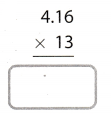We multiply as a whole number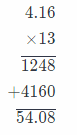Since there are two decimal digits in the factor 4.16, there must be two decimal digits in the product 54.08.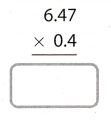We mutiply as a whole number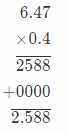Since there are two decimal digits in the factor 6.47 and one decimal digit in the factor 0.4, there must be three
decimal digits in the product 2.588.

Question 3.We multiply as a whole number.Since there are two decimal digits in the factor 7.05 and one decimal digit in the factor 9.4, there must be three
decimal digits in the product 66.270.

Question 4.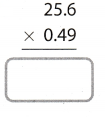We multiply as a whole number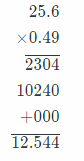Since there are two decimal digits in the factor 0.49 and one decimal digit in the factor 25.6, there must be three
decimal digits in the product 12.544

Area of Squares, Rectangles, and Triangles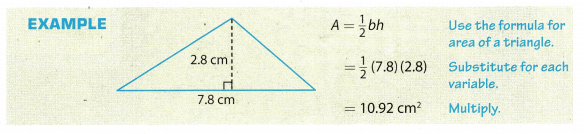Find the area of each figure.

triangle with a base of 14 in. and a height of 10 in. ____________________
The formula for the area of a triangle, where b is base and h is height is
A = $$\frac{1}{2}$$ b . h Substitute 14 in. for b, and 10 in. for h.
A = $$\frac{1}{2}$$ . 14 . 10
A = $$\frac{1}{2}$$ . 140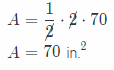Question 6.
square with sides of 3.5 ft . ___________
Formula for area of a square, where a represents side of a square, is
A = a2 Substitute 3.5 ft for a.
= (3.5)2
A = 12.25 ft2

Question 7.
rectangle with length 8$$\frac{1}{2}$$ in. and width 6 in. __________________
The formula for the area of a rectangle, where a represents length and b represents the width, is
A = a . b Substitute 8$$\frac{1}{2}$$ in. for a, and 6 in for b.
A = 8$$\frac{1}{2}$$ . 6
A = $$\frac{17}{2}$$ . 6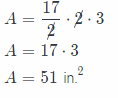Geometry Concepts and Applications Answer Key Pdf Question 8.
triangle with base 12.5 m and height 2.4 m _________________
The formula for the area of a triangle, where b is base and h is height is
A = $$\frac{1}{2}$$ . b . h Substitute 12.5 m for b, and 2.4 m for h.
A = $$\frac{1}{2}$$ . 12.5 . 2.4
A = $$\frac{1}{2}$$ . 30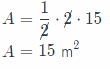Visualize Vocabulary

Use the ✓ words to complete the graphic. You will put one word in each oval.Understand Vocabulary

Complete each sentence, using the preview words.